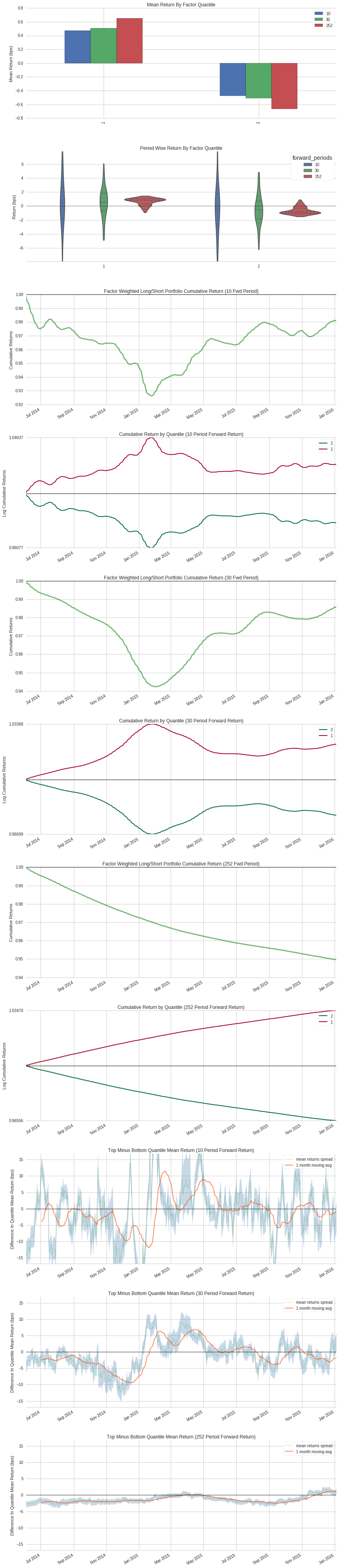Notebook

# Using Alphalens to study if forward_earning_yield has predictive power over returns¶

#### forward_earning_yield is defined here https://www.quantopian.com/help/fundamentals#valuation-ratios¶

Estimated Earnings Per Share / Price Note: a) The “Next” Year’s EPS Estimate is used; For instance, if today’s actual date is March 1, 2009, the “Current” EPS Estimate for MSFT is June 2009, and the “Next” EPS Estimate for MSFT is June 2010; the latter is used. b) The eps estimated data is sourced from a third party.

In :
from quantopian.research import run_pipeline, symbols
from quantopian.pipeline import Pipeline
from quantopian.pipeline.factors import Latest
from quantopian.pipeline.data.builtin import USEquityPricing
from quantopian.pipeline.data import morningstar
#from quantopian.pipeline.factors import CustomFactor, SimpleMovingAverage, AverageDollarVolume, Returns, RSI
#from quantopian.pipeline.classifiers.morningstar import Sector
from quantopian.pipeline.filters import Q500US, Q1500US
#from quantopian.pipeline.data.quandl import fred_usdontd156n as libor
#from quantopian.pipeline.data.zacks import EarningsSurprises

import pandas as pd
import numpy as np

import alphalens
#import pyfolio as pf
#from scipy import stats
#import matplotlib.pyplot as plt
#from sklearn import linear_model, decomposition, ensemble, preprocessing, isotonic, metrics

from quantopian.interactive.data.estimize import estimates_free
from quantopian.interactive.data.estimize import estimates
from quantopian.interactive.data.eventvestor import earnings_guidance_free
from quantopian.interactive.data.eventvestor import earnings_guidance

In :
universe = Q1500US()

def make_pipeline():

pipe = Pipeline(screen=universe,
columns={'close': close,
'forward_earning_yield': fey,
},
)
return pipe


In :
results = run_pipeline(make_pipeline(), '2014-01-01', '2017-01-01')
results.dropna(axis=0, inplace=True) ## drop days/stock pairs that have no forward_earning_yield

Out:
close forward_earning_yield
2014-06-04 00:00:00+00:00 Equity(2 [ARNC]) 13.65 0.0496
Equity(24 [AAPL]) 637.54 0.0790
Equity(41 [ARCB]) 42.03 0.0527
Equity(62 [ABT]) 39.82 0.0592

#### We have the data. All we need now is to reshape the data in a way that alphalens likes¶

Prepare factor

In :
factor = results['forward_earning_yield']

Out:
2014-06-04 00:00:00+00:00  Equity(2 [ARNC])     0.0496
Equity(24 [AAPL])    0.0790
Equity(41 [ARCB])    0.0527
Equity(62 [ABT])     0.0592
Name: forward_earning_yield, dtype: float64

Prepare prices

In :
prices = pd.DataFrame(results['close'])
prices = prices.unstack(level=1)
prices.dropna(axis=1, inplace=True) ## drop stocks with no forward_earning_yield
prices.columns = prices.columns.droplevel(0)

Out:
Equity(24 [AAPL]) Equity(62 [ABT]) Equity(67 [ADSK]) Equity(76 [TAP]) Equity(114 [ADBE]) Equity(122 [ADI]) Equity(128 [ADM]) Equity(161 [AEP]) Equity(166 [AES]) Equity(168 [AET]) ... Equity(45618 [AR]) Equity(45667 [VEEV]) Equity(45734 [COMM]) Equity(45755 [BRX]) Equity(45815 [TWTR]) Equity(45874 [ALLE]) Equity(45971 [AAL]) Equity(45993 [HLT]) Equity(46215 [SC]) Equity(46240 [RICE])
2014-06-04 00:00:00+00:00 637.54000 39.82 53.15 65.60 64.09 52.47 44.35 53.95 14.19 78.48 ... 63.055 20.05 24.52 22.10 32.58 52.42 41.44 22.70 20.090 32.580
2014-06-05 00:00:00+00:00 644.82000 39.74 53.03 65.57 64.17 52.49 44.31 54.00 13.98 79.40 ... 64.060 19.79 24.89 22.01 32.91 53.16 42.80 22.77 20.260 33.210
2014-06-06 00:00:00+00:00 647.35000 40.14 53.71 65.63 65.47 52.57 44.96 54.17 14.27 79.13 ... 63.500 20.75 24.41 22.24 33.88 54.30 42.41 23.02 20.310 33.315
2014-06-09 00:00:00+00:00 92.22613 40.05 54.87 66.72 66.92 52.69 45.19 54.05 14.45 79.47 ... 63.470 21.16 24.89 22.19 33.33 55.85 43.88 23.26 20.510 33.050
2014-06-10 00:00:00+00:00 93.70000 40.13 54.50 67.10 66.93 55.31 45.12 53.49 14.40 79.16 ... 62.220 21.49 25.00 21.87 34.46 56.35 43.57 23.28 20.615 32.610

5 rows × 1034 columns

#### Actually pass the data into alphalens¶

i use one large period (252 days) because forward_earning_yield is one-year forward looking

In :
factor_data = alphalens.utils.get_clean_factor_and_forward_returns(factor = factor,
prices = prices,
quantiles = 2,
#periods = (3, 10, 30),
periods = (10, 30, 252),
)


actually compute the tear sheet

In :
alphalens.tears.create_full_tear_sheet(factor_data)

Quantiles Statistics

min max mean std count count %
factor_quantile
1 -0.3609 0.0643 0.037361 0.024699 207210 50.09913
2 0.0551 3.2914 0.082043 0.035936 206390 49.90087
Returns Analysis

10 30 252
Ann. alpha -0.005 -0.006 -0.028
beta 0.019 -0.047 -0.236
Mean Period Wise Return Bottom Quantile (bps) 4.706 15.184 165.135
Mean Period Wise Spread (bps) -0.943 -1.014 -1.314
/usr/local/lib/python2.7/dist-packages/alphalens/plotting.py:727: FutureWarning: pd.rolling_apply is deprecated for Series and will be removed in a future version, replace with
Series.rolling(center=False,min_periods=1,window=10).apply(args=<tuple>,func=<function>,kwargs=<dict>)
min_periods=1, args=(period,))
/usr/local/lib/python2.7/dist-packages/alphalens/plotting.py:767: FutureWarning: pd.rolling_apply is deprecated for DataFrame and will be removed in a future version, replace with
DataFrame.rolling(center=False,min_periods=1,window=10).apply(args=<tuple>,func=<function>,kwargs=<dict>)
min_periods=1, args=(period,))
/usr/local/lib/python2.7/dist-packages/alphalens/plotting.py:727: FutureWarning: pd.rolling_apply is deprecated for Series and will be removed in a future version, replace with
Series.rolling(center=False,min_periods=1,window=30).apply(args=<tuple>,func=<function>,kwargs=<dict>)
min_periods=1, args=(period,))
/usr/local/lib/python2.7/dist-packages/alphalens/plotting.py:767: FutureWarning: pd.rolling_apply is deprecated for DataFrame and will be removed in a future version, replace with
DataFrame.rolling(center=False,min_periods=1,window=30).apply(args=<tuple>,func=<function>,kwargs=<dict>)
min_periods=1, args=(period,))
/usr/local/lib/python2.7/dist-packages/alphalens/plotting.py:727: FutureWarning: pd.rolling_apply is deprecated for Series and will be removed in a future version, replace with
Series.rolling(center=False,min_periods=1,window=252).apply(args=<tuple>,func=<function>,kwargs=<dict>)
min_periods=1, args=(period,))
/usr/local/lib/python2.7/dist-packages/alphalens/plotting.py:767: FutureWarning: pd.rolling_apply is deprecated for DataFrame and will be removed in a future version, replace with
DataFrame.rolling(center=False,min_periods=1,window=252).apply(args=<tuple>,func=<function>,kwargs=<dict>)
min_periods=1, args=(period,))
/usr/local/lib/python2.7/dist-packages/alphalens/plotting.py:519: FutureWarning: pd.rolling_mean is deprecated for Series and will be removed in a future version, replace with
Series.rolling(window=22,center=False).mean()

Information Analysis

10 30 252
IC Mean -0.010 -0.024 -0.069
IC Std. 0.097 0.088 0.054
t-stat(IC) -2.095 -5.387 -25.527
p-value(IC) 0.037 0.000 0.000
IC Skew 0.009 0.174 1.070
IC Kurtosis 0.115 0.325 0.820
Ann. IR -1.662 -4.276 -20.261
/usr/local/lib/python2.7/dist-packages/alphalens/plotting.py:215: FutureWarning: pd.rolling_mean is deprecated for Series and will be removed in a future version, replace with
Series.rolling(window=22,center=False).mean()
pd.rolling_mean(ic, 22).plot(ax=a,

Turnover Analysis

10 30 252
Quantile 1 Mean Turnover 0.042 0.077 0.196
Quantile 2 Mean Turnover 0.042 0.078 0.198
10 30 252
Mean Factor Rank Autocorrelation 0.984 0.957 0.761
<matplotlib.figure.Figure at 0x7f177c281910>